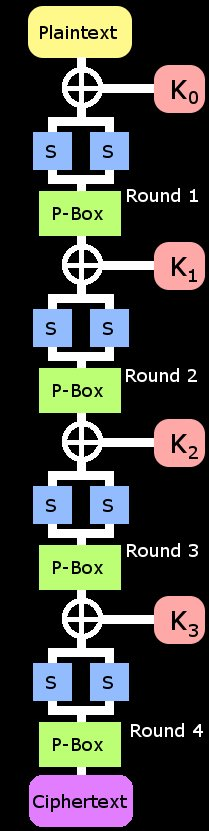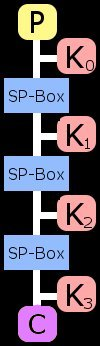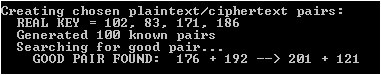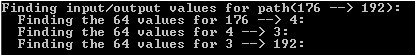# Multi-Round Differential Cryptanalysis

In this tutorial, we'll expand on the last one and use differential cryptanalysis to attack a much stronger block cipher. This one has 4 rounds; 3 of them effect the security. This means that using one good characteristic to find good pairs and eventually the key won't work. We need to chain differential characteristics together to get those guesses at the intermediate values in the structure of the cipher.

Source Code - This program, written in C, implements the toy cipher. It then calculates the most probable differential characteristics, displays all possible inputs/outputs for one selected characteristic path (176 -> 4 -> 3 -> 192), and recovers the key in much less time than brute force.

### Our Target SPN CipherThe first thing you'll notice about the new target is how long it is. It consists of 4 rounds each with its own subkey. The same substitution/permutation operation is repeated on the block along with the standard XOR key-mixing. The block size is 8 bits, the key is 32 bits, and the S-Boxes are 4-bits. The key is split into 4 subkeys each 8 bits in length. If we apply the trick learned in the last tutorial, you'll note that we do not need to brute force K3(the last subkey). We can use existing information and a known-pair to calculate it as the rest of the keyspace is exhaustively searched. This means that am exhaustive search would require testing 224 keys; that's right around 17 million!

In each round, the 8 bit block is XOR-mixed with the appropriate subkey. Next it is split into two halves; the most significant 4 bits become the left half and 4 least significant bits become the right. These halves are fed into the same 4 bit substitution box that was described in the last tutorial. The outputs are then combined back in the expected way to produce a substituted 8 bit block. I used two small S-Boxes instead of one large one mainly to keep things simple. Also there may be further breaks to explore with double S-Box setup versus one giant 8 bit box. Also, it means that I don't have generate and hardcode a giant 256-entry lookup table for it.

The last operation of the round takes the 8 bit output of the S-Box and feeds it into an 8 bit Permutation Box. This added element makes this cipher a true Substitution-Permutation Network(SPN). This P-Box simply takes bits from the input and feeds them to different bit positions in the output. This adds an element of diffusion meaning that it spreads the information around. In this case, it mixes the results of the two S-Boxes. If there were no P-Box, the block could be split in half and attacked 4 bits at a time. The permutation box ensures that this is not possible by mixing the output bits from the S-Boxes together.### Lets Simplify This ThingFirst thing's first; we're going to combine all of that double S-Box/P-Box stuff into a single function called SP-Box. This combined function takes an 8-bit input, runs it through the split substitution boxes and then through the P-Box to produce an 8-bit output. We'll be treating this big SP-Box function as an S-Box later when we search for differential characteristics. The second simplification we'll make is just like we did in the last tutorial. Because the last substitution box is reversible, we can ignore it; it does not affect the security of the cipher. Also remember that the last subkey can be found if we correctly guess the other subkeys.

So, in our simplified version of the algorithm, the input is mixed with K0 then ran through the SP-Box. Next, the block is mixed with K1 and ran through the SP-Box again. The block is then mixed with K2 and SP-Boxed yet again. Finally, the data is XOR-mixed with the last subkey K3. Note that every operation used in this simplified cipher is 8-bit. This will keep things simple as we build a differential attack against it.

### Finding a Characteristic Path

Next we write a program that will search all combinations of inputs to the new SP-Box and keep track of what characteristics this produces. This will build a 256x256 table with entries that tells us how many input pairs produce each differential characteristic. An entry will a zero value implies that the input->output characteristic never occurs. We filter this table and display the highest-valued differential characteristics. This means that we're only interested in the characteristics most likely to occur. We find several entries that hold for 96/256 pairs and many that hold for 64/256.The next thing we must do is search this filtered list for 3-link chains of characteristics. I did this searching by hand, but I'm sure there is a way to automate it as well. Look for differential pairs that lead to other differential pairs. For example, the differential path I found was: "176 --> 4 --> 3 --> 192". The first characteristic in this path is "176 --> 4" and it held true 64/256. This means that when we tested 256 input pairs with an input differential of 176, the SP-Box produced an output differential of 4 for exactly 64 of them. The next characteristic in the path is "4 --> 3" which held true 64/256 as well. Notice that the output differential of the first characteristic matches the input differential of the second. We chain them together like this because the only time the differential changes as a good pair flows through the cipher is when it hits the SP-Box. The final characteristic in the path is "3 --> 192" which holds true for 64/256 pairs as well.

All of this means that if we feed the cipher a chosen plaintext/ciphertext pair whose plaintexts have a differential of 176, there is reasonable probability that the ciphertexts' differential will be 192.

### Search for a Good Pair

Next gather some plaintext/ciphertext pairs. These will be used to test if our key guesses are correct. This portion of the attack only needs to be known-plaintext rather than chosen-plaintext. Because the cipher encrypts an 8-bit block using a 32-bit key; there will be many decoy keys that will be correct for one tested pair but not others. For this reason, you should gather a decent amount of plaintext/ciphertext pairs for validation.

Now we begin the search for a good pair. Choose a random plaintext block and call it P0. Next XOR P0 with the input to your differential path (176 in our case) to produce P1. Now run both of these chosen-plaintexts through the cipher to produce their cooresponding ciphertexts C0 and C1. Now XOR these ciphertexts together to get the output differential of the chosen-pair. If it matches the output differential of your path (192 in our case), you have found a good pair. Continue choosing pairs until you find a good one for your differential characteristic path through the cipher. You can also save the "bad" pairs for use in validating key guesses.If you find a good pair, proceed as if it occured as your path describes. We can only know the plaintext and ciphertext differentials in this multi-round cipher. The differentials in the middle are a mystery to us. But we proceed as if the ciphertext differential was created via the same route as our path describes. So assume that these intermediate differentials inside the cipher match those described by our path. There is a very real possibility that the differential was generated by another path; but we assembled ours because it was likely to occur. The diagram in the center of the page above shows what the assumed differentials are in between the SP-Boxes in our example (when applied to a good pair).

### Recover the Key

Once you've chosen a differential path through the cipher, you should record all of the input/output pairs that produce each characteristic in that path. There are 64 of these pairs for each in our example. This suggests that the inputs and outputs for each SP-Box are limited to these 64 pre-known values. All that's left to do is use these possible known intermediate values to calculate a guess at each of the subkeys. Also use the known plaintext/ciphertext of the good pair in this effort.By looping through all of the possible values that could have produced each characteristic displayed by the good pair, we have limited the amount of keyspace that must be searched. We try all 64 possibilities of inputs to SP-Box #1 (for "176 --> 4") against all 64 for SP-Box #2 (for "4 --> 3") against all 64 for SP-Box #3 (for "3 --> 192"). This means that after all of this precomputation madness is over and we have a good pair in hand; we only need to test 64 * 64 * 64 keys to recover the correct one. Of course, each guess is validated against our collection of known-plaintext/ciphertext. This work effort is much smaller than the brute force alternative: 224.Remember that there are multiple paths that the differential could have taken to arrive at a "good pair". Any path that goes from "176 --> 192" may have created the good pair we found. My testing showed that the differential followed the path described above roughly 25% of the time. When it did, the key was recovered. In order to recover the key 25% of the time via brute force, we'd have to try around 4 million keys. Using this attack, we find it 25% of the time by testing only 260,000 keys. Also, it should be noted that this 25% figure includes instances where a good pair was not found at all. By trying 256 chosen-pairs, a "good" one was found around 80% of the time. For bonus points try finding a different path that includes 96/256 or two and see how it affects these results.

### Fun Stuff

This tutorial moved a little faster than the last one. I skimmed over the actual math involved in finding the subkeys based on those 64 possibilities per SP-Box. Check out the code for the details on that; or better yet try to find it yourself. Refer back to the Differential Cryptanalysis Tutorial if things are getting confusing. Also, shoot me an email if you made it through this one and let me know what you think.